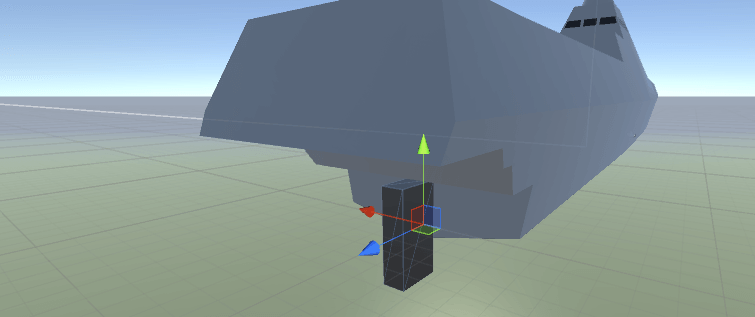# Make a realistic boat in Unity with C#

Alright we are inching our way towards a boat. The boat can currently float, but it also has to move, so we need to add an engine and a propeller.

Adding a propeller is just a matter of adding a force where the propeller is, which will make the boat move forward. But it also has to turn. But I'm lucky because I'm modelling a boat that's propelled forward not with a propeller but with a water jet. This water jet will also steer the boat by turning the nozzle in another direction. If you have a rudder you have to find another solution!

This is how my BoatEngine script looks like (just add it to the boat you have):

```using UnityEngine;
using System.Collections;

public class BoatEngine : MonoBehaviour
{
//Drags
public Transform waterJetTransform;

//How fast should the engine accelerate?
public float powerFactor;

//What's the boat's maximum engine power?
public float maxPower;

//The boat's current engine power is public for debugging
public float currentJetPower;

private float thrustFromWaterJet = 0f;

private Rigidbody boatRB;

private float WaterJetRotation_Y = 0f;

BoatController boatController;

void Start()
{
boatRB = GetComponent<Rigidbody>();

boatController = GetComponent<BoatController>();
}

void Update()
{
UserInput();
}

void FixedUpdate()
{
UpdateWaterJet();
}

void UserInput()
{
//Forward / reverse
if (Input.GetKey(KeyCode.W))
{
if (boatController.CurrentSpeed < 50f && currentJetPower < maxPower)
{
currentJetPower += 1f * powerFactor;
}
}
else
{
currentJetPower = 0f;
}

//Steer left
if (Input.GetKey(KeyCode.A))
{
WaterJetRotation_Y = waterJetTransform.localEulerAngles.y + 2f;

if (WaterJetRotation_Y > 30f && WaterJetRotation_Y < 270f)
{
WaterJetRotation_Y = 30f;
}

Vector3 newRotation = new Vector3(0f, WaterJetRotation_Y, 0f);

waterJetTransform.localEulerAngles = newRotation;
}
//Steer right
else if (Input.GetKey(KeyCode.D))
{
WaterJetRotation_Y = waterJetTransform.localEulerAngles.y - 2f;

if (WaterJetRotation_Y < 330f && WaterJetRotation_Y > 90f)
{
WaterJetRotation_Y = 330f;
}

Vector3 newRotation = new Vector3(0f, WaterJetRotation_Y, 0f);

waterJetTransform.localEulerAngles = newRotation;
}
}

void UpdateWaterJet()
{
//Debug.Log(boatController.CurrentSpeed);

Vector3 forceToAdd = -waterJetTransform.forward * currentJetPower;

//Only add the force if the engine is below sea level
float waveYPos = WaterController.current.GetWaveYPos(waterJetTransform.position, Time.time);

if (waterJetTransform.position.y < waveYPos)
{
}
else
{
}
}
}
```

To make the script work you have to tell it where the water jet is by giving it a gameobject. This gameobject is just an empty gameobject which is a child to the boat and its position is where the waterjet is. You can also add a box so you can see in which way the water jet is pointing.You also need a way to calculate the speed. And I've added the speed calculations to another script called BoatController.

```using UnityEngine;
using System.Collections;

public class BoatController : MonoBehaviour
{
//Speed calculations
private float currentSpeed;
private Vector3 lastPosition;

void FixedUpdate()
{
CalculateSpeed();

//Debug.Log(currentSpeed);
}

//Calculate the current speed in m/s
private void CalculateSpeed()
{
//Calculate the distance of the Transform Object between the fixedupdate calls with
//'(transform.position - lastPosition).magnitude' Now you know the 'meter per fixedupdate'
//Divide this value by Time.deltaTime to get meter per second
currentSpeed = (transform.position - lastPosition).magnitude / Time.deltaTime;

//Save the position for the next update
lastPosition = transform.position;
}

public float CurrentSpeed
{
get
{
return this.currentSpeed;
}
}
}
```

And now you have your very own boat!